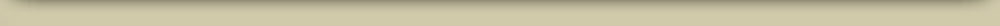# Courses Central to IDEATE

The following four graduate courses cover topics central to the electric drivetrain. They address: battery modeling, design of battery management systems (BMS), vehicle power electronics, and motor drive.

All courses are three-credit-hours each. If you qualify for in-state tuition, then regular in-state tuition rates apply. If you do not qualify for in-state tuition, the 2018-2019 rates for tuition plus fees are \$2,152 per course at UCCS. The rates for courses from CU-Boulder are posted here. A limited number of Department-of-Energy sponsored GATE Fellowships are available, to assist with tuition, to well-qualified students taking IDEATE courses at the CU-Boulder campus.

### ECE 5710: Modeling, Simulation, and Identification of Battery Dynamics (UCCS)

This course derives mathematical models of the electrochemical dynamics of battery cells, including thermodynamic and kinematic properties, at multiple scales. Modern, lithium-ion chemistries are emphasized. Students use simulation software and lab-test data to create and validate parameterized models. Topics of the course include:
1. Battery boot camp.
2. Equivalent-circuit cell models.
3. Microscale cell models.
4. Continuum (porous electrode) cell models.
5. State-space models and the dynamic realization algorithm.
6. Reduced-order models of cell dynamics.
7. Thermal models.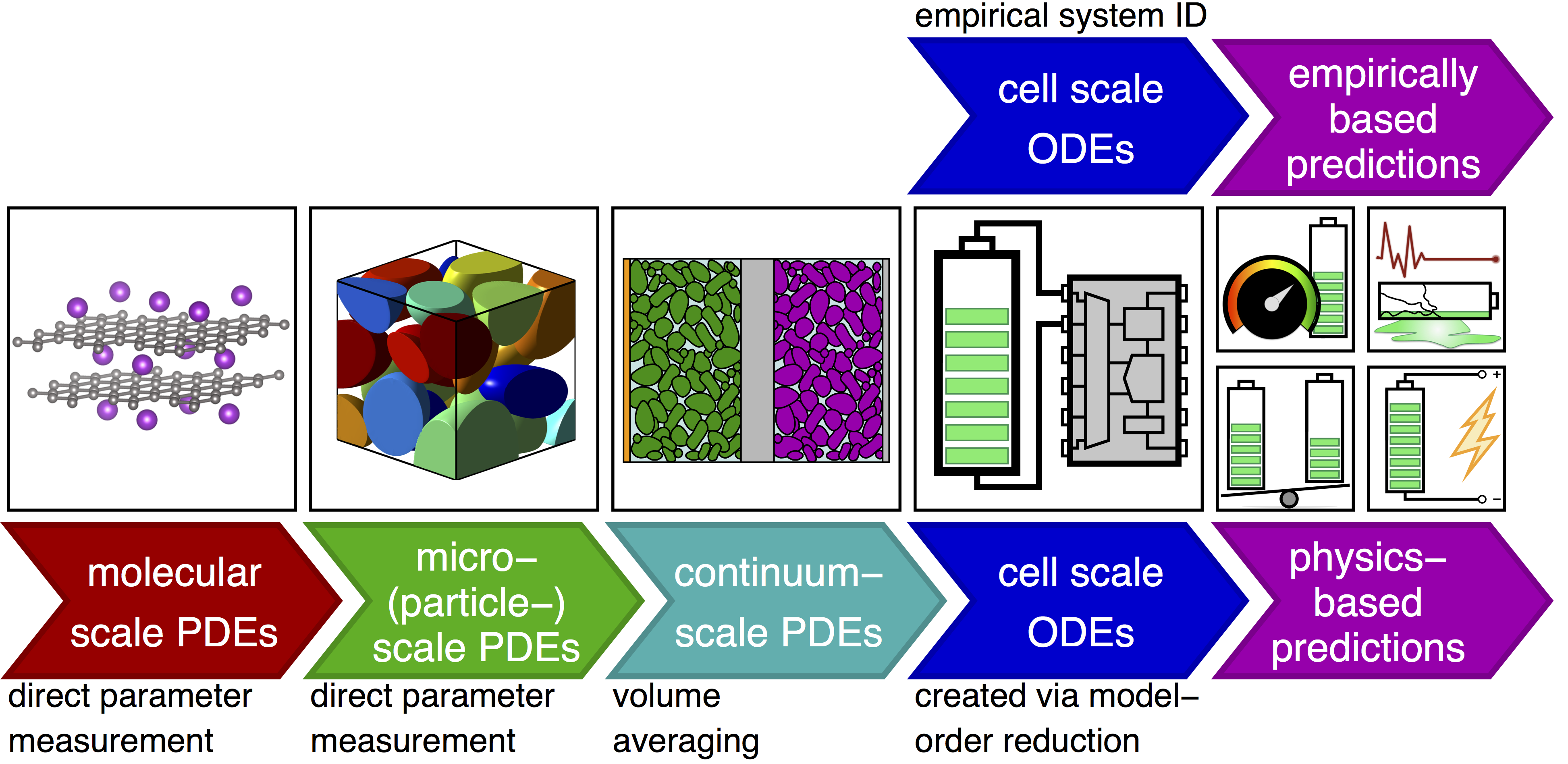### ECE 5720: Battery Management and Control (UCCS)

This course considers the design of battery management systems. Basic thermal and high-voltage electrical control are considered. Architectures for modular design are compared and contrasted. Different methods for cell equalization are introduced. Algorithms for estimating state-of-charge, state-of-health, and state-of-life of a battery pack are studied in depth. Students will design battery management system routines for state-of-charge and state-of-health estimation, will implement, test, and validate the algorithms on laboratory-generated data. Topics of the course include:
1. Functions of a battery management system.
2. Battery models and simulation of battery packs.
3. Battery state estimation.
4. Battery health estimation.
5. Cell balancing.
6. Voltage-based power limit estimation.
7. Aging mechanisms and degradation models.
8. Optimized controls for power estimation.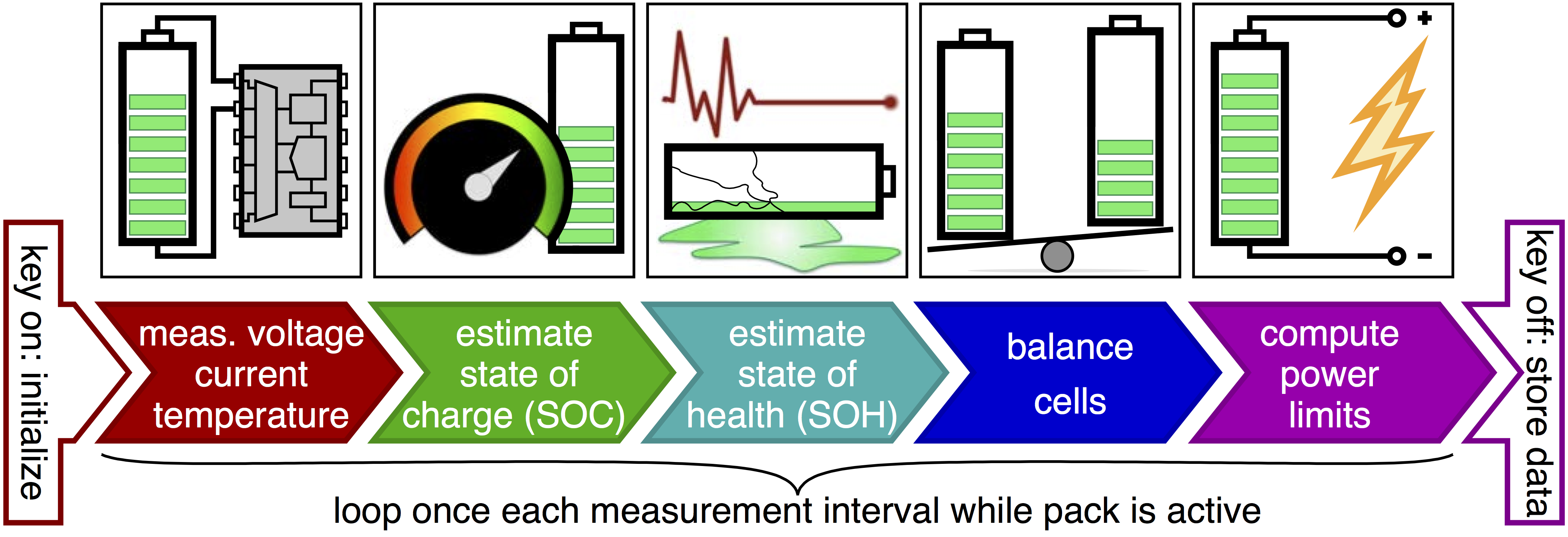### ECEN 5017: Power Electronics for Electric Drive Vehicles (CU-Boulder)

This course considers the design and control of power converters in electric drive vehicles. The course includes an overview of system architectures and covers system-level dynamic modeling and control using Simulink at levels appropriate to determine requirements and validate the performance of power converters in the vehicle system. Topics of the course include:

1. Electric drive vehicle system architecture.
2. Electric system control and dynamic modeling in Simulink.
3. Power converters for motor drives.
4. Power converters for energy storage, battery managment electronics.
5. Power converters for battery chargers and utility interface.
Link to course materials at CU-Boulder.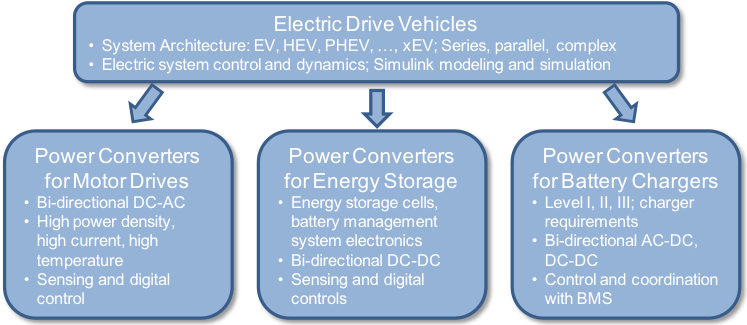### ECEN 5737: Adjustable Speed AC Drives (CU-Boulder)

This course considers the design of motor drives for electric vehicles. The course covers basic principles for analysis of electric machines and reference frame theory and develops in detail the operation and control algorithms for symmetrical induction machines and permanent magnet synchronous machines. Emphasis is placed on practical design for the electric vehicle application. Topics of the course include:
1. Introduction to electric machines for electric vehicles.
2. Principles for analysis of electric machines, reference frame theory.
3. Operation and control of symmetrical induction machines.
4. Operation and control of permanent-magnet synchronous machines.
Link to course materials at CU-Boulder.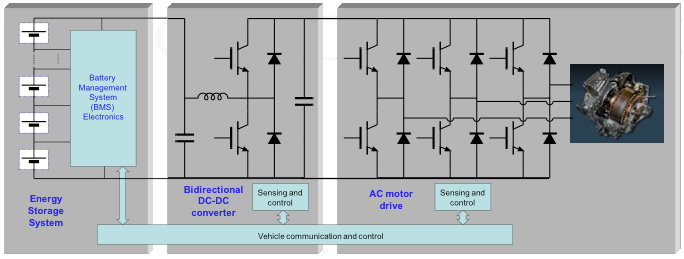# Additional Courses Related to IDEATE at UCCS

### ECE 5510: Feedback Control Systems

Linear analysis and analog simulation of electrical, chemical, hydraulic, and mechanical systems using block diagrams and signal-flow graphs. Comparison of open- and closed-loop configurations. Feedback control system design using Nyquist, Bode, and root-locus methods. Effects of simple networks on system response. Introduction of state-variable techniques and digital computer solutions.
1. Introduction to feedback control.
2. System modeling in the time domain.
3. Dynamic response.
4. Basic properties of feedback.
5. Stability analysis.
6. Root-locus analysis.
7. Root-locus design.
8. Frequency-response analysis.
9. Frequency-response design.
10. Digital control systems.

### ECE 5520: Multivariable Control Systems I

Fundamental aspects of modern control theory are covered, including solutions to systems modeled in state-variable format, controllability, observability, pole placement, and linear transformation. Computer based tools for control system design are used.
1. Linear algebra (matrix) review.
2. Continuous-time state-space systems.
3. Discrete-time state-space systems.
4. Observability and controllability.
5. Controllers, observers and compensators.
6. Introduction to linear quadratic regulation.

### ECE 5530: Multivariable Control Systems II

Design of systems in state variable format are covered including linear quadratic regulators, state estimators, model-reference compensators, and H control. Computer tools are used.
1. Vector random processes.
2. Performance.
3. Linear quadratic regulator.
4. Least-squares estimation.
5. Optimal Kalman filtering and state estimation.
6. Linear quadratic Gaussian control.
7. Introduction to robust control.
8. H full-information control and estimation.
9. H output feedback.

### ECE 5540: Digital Control Systems

Theory and application of classical and modern discrete-time control systems. Analysis and design of discrete-time and hybrid control using z-transforms, root locus, frequency domain and state vari- able compensation techniques. On-line implementation by digital computers will be studied.
1. Introduction to Digital Control.
2. Emulation of Analog Controllers (I).
3. The z-Transform.
4. Emulation of Analog Controllers (II).
5. Sampling and Reconstruction.
6. Discrete-Time Systems.
7. Stability Analysis Techniques.
8. Digital Controller Design.
9. Digital Filter Structures and Quantization Effects.
10. Adaptive Inverse Control.

### ECE 5550: Applied Kalman Filtering

Theory and application of Kalman filters for state estimation, information fusion, multitarget tracking, and data association. Special focus on the discrete linear Kalman filter, the extended Kalman filter, and the unscented Kalman filter. Practical issues related to robust performance are studied.
1. Introduction to Kalman filters.
2. State-space dynamic systems.
3. Dynamic systems with noisy inputs.
4. The linear Kalman filter.
5. Practical Kalman filtering.
6. IMM/multitarget tracking.
7. Nonlinear Kalman filters.
8. Simultaneous state and parameter estimation.
9. Kalman filter applications.

### ECE 5560: System Identification

Modern methods for identifying mathematical models of systems from observations of their behavior; input-output and state-space models; parameterization and identifiability; non-parametric methods; prediction and output error methods; recursive estimation; Kalman filters; order estimation; subspace identification.
1. Introduction to system identification.
2. LTI systems, time-domain nonparametric system ID.
3. Frequency-domain nonparametric system ID.
4. Transfer function models and parametric system ID.
5. Deterministic state space models and system ID.
6. Stochastic state space models and system ID.
7. Feedback and real-time system ID.

### ECE 5570: Optimization Methods for Systems and Control

Optimization methods: parameter optimization, interior point methods, quadratic programming, constrained optimization, optimization for dynamic systems, optimal control and numerical methods. Engineering applications, especially control.
1. Review of linear algebra and vector calculus.
2. Parameter optimization: single-variable and multi-variable.
3. Parameter optimization: unconstrained and constrained.
4. Linear and quadratic optimization.
5. Convex optimization.
6. Optimization for dynamic systems: discrete-time and continuous-time.
7. Linear quadratic optimal control.

### ECE 5580: Multivariable Control Systems: Analysis and Design in Frequency Domain

This course teaches practical feedback control for linear multivariable systems from a frequency domain perspective. The focus is on developing useful techniques for the analysis and design of control systems for multiple-input-multiple-output (MIMO) plants from a classical control perspective and understanding their fundamental limitations. The course treats uncertainty as an important aspect of feedback design and incorporates methods for addressing robust stability and performance.
1. Review of linear algebra and functions of complex variables.
2. Classical feedback control techniques: frequency response and loop shaping.
3. Limitations on performance in SISO systems.
4. Uncertainty and robustness for SISO systems.
5. Linear systems theory for transfer matrix representations.
6. Multivariable frequency response analysis: characteristic loci and principle gains.
7. Multivariable control techniques.
8. Robust stability and performance analysis for MIMO systems.
9. MIMO controller design.
10. H-infinity control.
11. Model reduction.

### ECE 5590: Model Predictive Control

This course addresses the design of model predictive controllers for linear, time-invariant systems with constraints described by discrete-time state-space models. It develops the underlying principles of predictive control and highlights relationships to existing control theory.
1. Review of mathematical fundamentals.
2. Dynamic systems.
3. Discrete-time systems.
4. Model predictive control formulation.
5. Discrete-time model predictive control with constraints.
6. Stability.
7. Robust model predictive control.
8. Case study examples and applications of model predictive control.

# Additional Courses Related to IDEATE at CU-Boulder

### ECEN 5138: Control Systems Analysis

Analysis and design of continuous time control systems using classical and state space methods. Laplace transforms, transfer functions and block diagrams. Stability, dynamic response, and steady-state analysis. Analysis and design of control systems using root locus and frequency response methods. Computer aided design and analysis. Topics include:

1. What is control? History and examples, plants, controllers, and block diagrams; Why use feedback? Basic ideas
2. Review: ODE's, convolution, impulse response, Laplace transform, and transfer functions
3. Modeling, Newton’s laws, Lagrange formulation, Differential and s-domain models of mechanical, electrical, electromechanical, thermal, and fluidic systems
4. Dynamic models and dynamic response in terms of s-domain specifications
5. Block diagram manipulation and simplification
6. Basic feedback loop and important closed-loop maps including sensitivity and complementary sensitivity
7. Poles, zeroes and associated time responses, damping ratios, internal and external stability, final value theorem
8. Simple feedback types (P,D,PI,PD,PID) and their rule of thumb effects
9. Routh stability criterion, root locus analysis and design, steady-state response, bandwidth, tracking and system type, interplay between bandwidth and rise time, lead, lag and lead/lag design
10. Nyquist theorem, gain and phase margins
11. State feedback and pole placement, observers and observer based controllers, sensitivity

### ECEN 5458: Sampled Data and Digital Control Systems

This course covers how to analyze sampled-data systems (mixed discrete-time and continuous-time systems) and how to design practical digital controllers and analyze their performance using both frequency-domain (root locus, Bode, Nyquist) and time-domain (state-space) methods. Studies sampling theorem and sampling process characterization, z-transform theory and z-transfer function, and stability theory. Involves data converters (A/D and D/A), dead-beat design, and digital controller design. Topics include:
1. Analysis and design of continuous time control systems using classical and state space methods
2. Laplace transforms, transfer functions and block diagrams
3. Stability, dynamic response, and steady-state analysis
4. Analysis and design of control systems using root locus and frequency response methods
5. Computer aided design and analysis

### ECEN 5517: Power Electronics and Photovoltaic Power Systems Laboratory

This course is an introduction to analysis, modeling, design, and testing of electrical energy processing systems, in a practical laboratory setting. Included are power electronics converters for efficient utilization of available energy sources, and energy sources including solar panels and the utility. The semester lab work involves design, fabrication, and testing of a solar power system. Experiments covered:
1. Basic PV system elements (1 week)
2. Microcontroller system based on the TI MSP 430 (1 week)
3. Peak power tracker and battery charge controller using a dc-dc buck converter (4 weeks)
4. Inverter (4 weeks)
5. Project (4 weeks)

### ECEN 5613: Embedded System Design

Introduces system hardware and firmware design for embedded applications. Students independently design and develop a hardware platform encompassing a microcontroller and peripherals. Firmware is developed in C and assembly. A significant final project is designed, developed, documented, and presented. Topics include:

1. Introduction to embedded systems, design cycle, planning a development project
2. Microprocessor/microcontroller architectures and instruction sets, data buses, bus architectures
3. Assembly code, device programmers
4. Schematics, board layouts, manufacturing and test engineering, debugging strategies
5. Peripherals, timing diagrams and requirements
6. Memory selection, interface, maps
7. Serial communication
8. Assemblers, compilers, software tools
9. Interrupts in embedded C
10. Data converters
11. Final project

### ECEN 5797: Introduction to Power Electronics

An introduction to switched-mode dc-dc converters. The first part of the course treats basic circuit operation, including steady-state converter modeling and analysis, switch realization, discontinuous conduction mode, and transformer-isolated converters. Next, converter control systems are covered, including ac modeling of converters using averaged methods, small-signal transfer functions, and classical feedback loop design. Finally, magnetics design for switched-mode applications is discussed, including: basic magnetics, the skin and proximity effects, inductor design, transformer design. Topics include:

1. Introduction to power processing, applications and elements of power electronics
2. Principles of steady-state analysis
3. Steady-state equivalent circuit modeling, losses, and efficiency
4. Switch realization
5. The discontinuous conduction mode
6. Converter circuits
7. AC equivalent circuit modeling
8. Converter transfer functions
9. Controller design
10. Magnetics design

### ECEN 5807: Modeling and Control of Power Electronic Systems

Studies modeling and control topics in power electronics. Averaged switch modeling of converters, computer simulation, AC modeling of the discontinuous conduction mode, the current programmed mode, null double injection techniques in linear circuits, input filter design, and low-harmonic rectifiers. Topics include:
1. Averaged switch modeling and simulation
2. Techniques of design-oriented analysis
3. Dynamic modeling and simulation of converters operating in discontinuous conduction mode
4. Introduction to sampled-data modeling
5. Current programmed control
6. Introduction to digital control of switching converters
7. Modern rectifiers

### ECEN 5817: Resonant and Soft-Switching Techniques in Power Electronics

The course includes a detailed study of the mechanisms of switching loss, hard switching, and soft switching. Both traditional resonant converters and up-to-date approaches to soft switching are included. Applications emphasized include dc-dc converters for computer power and portable applications, and dc-ac inverters for gas discharge lighting. Analysis approaches include steady-state analysis in the frequency domain (using sinusoidal approximation) and time domain (state plane analysis), and small-signal ac modeling using the averaged switch modeling and the phasor transform methods. Topics include optimization of system efficiency at both full- and light-load conditions for improved energy efficiency. Topics include:
1. Introduction to resonant converter applications and approaches
2. Sinusoidal analysis in steady-state
3. Sinusoidal analysis: small-signal ac behaviour with frequency modulation
4. State-plan analysis of resonant and soft-switching converters
5. Resonant switch and related converters
6. Server systems, portable power, energy efficiency and green power issues

### ECEN 5827: Analog IC Design

Covers the fundamentals of transistor-level analog integrated circuit design. Starting with motivations from application circuits, the course develops principles of DC biasing, device models, amplifier stages, frequency response analysis and feedback and compensation techniques for multi-stage operational amplifiers. Topics include:
1. Op-amp application circuits and op-amp characteristics
2. Transistor-level view of a two-stage op-amp
3. Introduction to negative feedback circuits
4. Reference circuits and voltage regulators
5. Device high-frequency small-signal models & capacitances
6. Frequency-response and stability of feedback circuits
7. Examples of analysis and design of more advanced analog building blocks

### ECEN 5837: Mixed Signal IC Design Lab

Software laboratory course extends the concepts developed in ECEN 5827 to full design and layout of mixed analog and digital custom integrated circuits. Assignments explore implementation of analog to digital and digital to analog converters, and final project develops a full custom IC for a target application. Topics include:
1. Introduction to system-on-a-chip and mixed-signal IC design
2. Introduction to IC layout and fabrication processes
3. Co-design of analog and digital components, software tools
4. Fully differential op-amps and comparators
5. Switched capacitor circuits
6. Design of digital to analog converters
7. Design of analog to digital converters
8. Final project: complete mixed-signal IC design